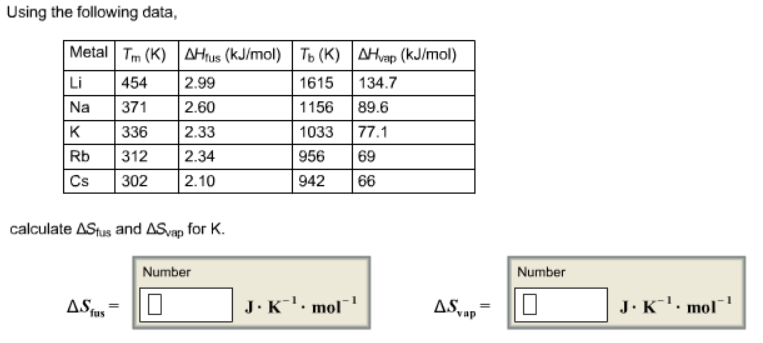# Problem: Using the following data, calculate ΔSfus and ΔSvap for K.

###### FREE Expert Solution

We are being asked to calculate ΔSfus and ΔSvap for K

Recall the equation

We are given

ΔHfus K at 336 K = 2.33 kJ/mol

ΔHvap K at 1033 K = 77.1 kJ/mol

81% (45 ratings)###### Problem Details

Using the following data, calculate ΔSfus and ΔSvap for K.Frequently Asked Questions

What scientific concept do you need to know in order to solve this problem?

Our tutors have indicated that to solve this problem you will need to apply the Entropy concept. You can view video lessons to learn Entropy. Or if you need more Entropy practice, you can also practice Entropy practice problems.